# Welcome to the Logics and Proofs MCQs Page

Dive deep into the fascinating world of Logics and Proofs with our comprehensive set of Multiple-Choice Questions (MCQs). This page is dedicated to exploring the fundamental concepts and intricacies of Logics and Proofs, a crucial aspect of Discrete Mathematics. In this section, you will encounter a diverse range of MCQs that cover various aspects of Logics and Proofs, from the basic principles to advanced topics. Each question is thoughtfully crafted to challenge your knowledge and deepen your understanding of this critical subcategory within Discrete Mathematics.

Check out the MCQs below to embark on an enriching journey through Logics and Proofs. Test your knowledge, expand your horizons, and solidify your grasp on this vital area of Discrete Mathematics.

Note: Each MCQ comes with multiple answer choices. Select the most appropriate option and test your understanding of Logics and Proofs. You can click on an option to test your knowledge before viewing the solution for a MCQ. Happy learning!

### Logics and Proofs MCQs | Page 1 of 12

Q1.
In the circuit shown the lamp will be glowing if _________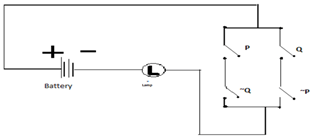Q2.
In this circuit shown the lamp will be glowing if _________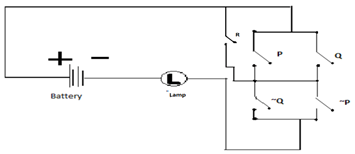Answer: (c).P: False, Q: False, R: True
Q3.
Which statement should be true in order for lamp to glow?Answer: (a).(R ∧ (~(P ∧ Q))
Q4.
If it is given that switch R is closed and Q is closed then the lamp will glow if _________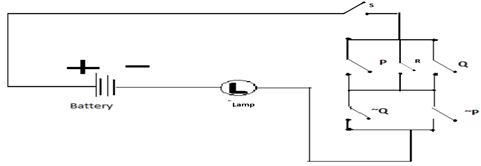Q5.
The circuit depend on which switch/switches state to be complete?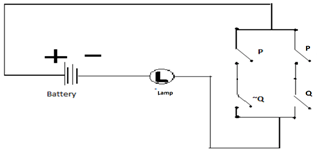a.

P

b.

Q

Q6.
The ten switches A,B,C,D,E,F,G,H,N,M are placed in the given circuit (all are open at given time). If you close one switch you need to pay 1 unit cost. What is the cost you need to pay to glow this Lamp?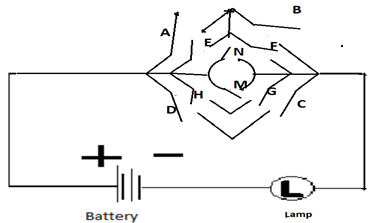Q7.
The given circuit can work if the switches P and Q be ___________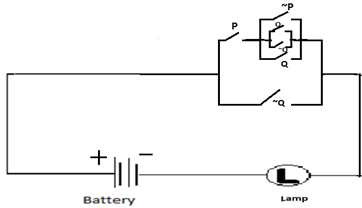Q8.
Which of the following statement is a proposition?
Answer: (d).The only odd prime number is 2
Q9.
The truth value of ‘4+3=7 or 5 is not prime’.